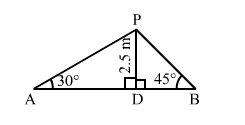# From a point on a bridge across a river, the angles of depression of the banks on opposite sides of the river are 30° and 45° respectively.`
Question:

From a point on a bridge across a river, the angles of depression of the banks on opposite sides of the river are 30° and 45° respectively. If the bridge is at a height of 2.5 m from the banks, find width of the river.

Solution:Let $A$ and $B$ be two points on the banks on the opposite side of the river and $P$ be the point on the bridge at a height of $2.5 \mathrm{~m}$.

Thus, we have:

$D P=2.5 \mathrm{~m}, \angle P A D=30^{\circ}$ and $\angle P B D=45^{\circ}$

In the right $\triangle A P D$, we have:

$\frac{D P}{A D}=\tan 30^{\circ}=\frac{1}{\sqrt{3}}$

$\Rightarrow \frac{2.5}{A D}=\frac{1}{\sqrt{3}}$

$\Rightarrow A D=2.5 \sqrt{3} \mathrm{~m}$

In the right $\Delta P D B$, we have:

$\frac{D P}{B D}=\tan 45^{\circ}=1$

$\Rightarrow \frac{2.5}{B D}=1$

$\Rightarrow B D=2.5 \mathrm{~m}$

$\therefore$ Width of the river $=A B=(A D+B D)=(2.5 \sqrt{3}+2.5)=6.83 \mathrm{~m}$